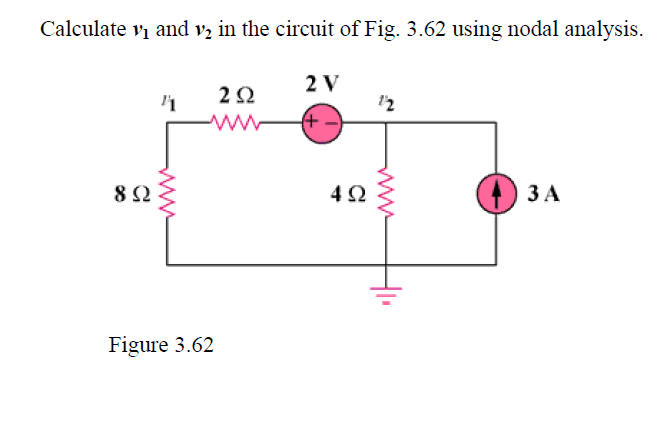# Exploring the Role of Batteries in Nodal Analysis

• goonking
Always mark up the circuit with arrows defining what you mean by +ve current. It does NOT matter which direction you choose for these.Then you need to mark up the circuit with arrows defining what you mean by +ve voltage across components like resistors. This must be consistent with the direction of the currents you defined above.If you follow this method there is no need to try and figure out in advance which way current will flow in any component at the outset. In some circuits that is next to impossible anyway. Using this method that will all come out in the wash. The sign of the answer and a quick look back at your marked up drawing will tell you.

## Homework Statement## The Attempt at a Solution

If I take KCL at node V1 (I'm assuming current traveling from nodeV2 to node V1)

Current in = Current out

V2 - 2volts / 2 ohms = V1 / 8 ohms

so my question is, how does the battery (2 volts in this case) play a role in nodal analysis? If I assume current enters through the negative terminal of the battery and out the poistive, it would be V2 - 2 volts / 2 ohms, correct? And V2 + 2 volts / 2 ohms if I assume current to go from V1 to V2.

is it possible to create a supernode here?

Your equation for the "current in" is incorrect. The voltage across the 2Ohm is not "V2-2volts". It's not "V2+2volts" either

CWatters said:
Your equation for the "current in" is incorrect. The voltage across the 2Ohm is not "V2-2volts". It's not "V2+2volts" either
how does the battery affect the voltage of the two nodes?
does it mean the voltage on Node1 is 2 volts higher than node2?

goonking said:
how does the battery affect the voltage of the two nodes?
does it mean the voltage on Node1 is 2 volts higher than node2?
Battery adds a p.d. of 2V along the path from V2 to V1, but there's also a voltage drop across the 2Ω resistance.

cnh1995 said:
Battery adds a p.d. of 2V along the path from V2 to V1, but there's also a voltage drop across the 2Ω resistance.
so that means V1 - V2 = 2volts difference?

and since current flows from higher voltage to lower, the current is actually going from V1 to V2?

goonking said:
so that means V1 - V2 = 2volts difference?
No. V1=V2+2V-I*(2Ω).
Conventional current direction is from V2 to V1, since conventional current flows from +ve terminal of the battery to the negative terminal.

•goonking
cnh1995 said:
No. V1=V2+2V-I*(2Ω).
Conventional current direction is from V2 to V1, since conventional current flows from +ve terminal of the battery to the negative terminal.
Oh, i see, using KVL

Correct.

When applying KVC and KVL there are some golden rules...

Always mark up the circuit at the outset with arrows defining what you mean by +ve current. It does NOT matter which direction you choose for these.

Then you need to mark up the circuit with arrows defining what you mean by +ve voltage across components like resistors. This must be consistent with the direction of the currents you defined above.

I repeat... It does not matter which direction you define as +ve current as long as these two steps are consistent with each other. Then later when you solve the equations if a voltage of current turns out to be -ve then you can refer back to the drawing to see what that means in practice.

If you follow this method there is no need to try and figure out in advance which way current will flow in any component at the outset. In some circuits that is next to impossible anyway. Using this method that will all come out in the wash. The sign of the answer and a quick look back at your marked up drawing will tell you.